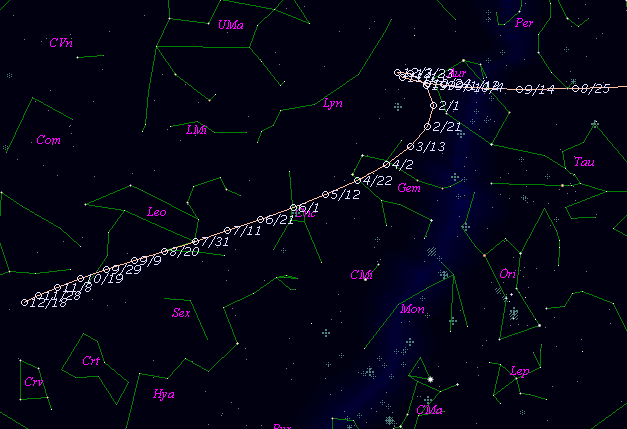# 93P/Lovas 1 (1998)###Pictures###Orbital Elements

```   The following improved orbital elements by Kenji Muraoka,
are from 117 observations 1980 to 1998, including 9 Planets,
Moon and 5 minor planets perturbations and non-gravitational
effect of style II.  The mean residual is +/- 0.70 arc seconds.

Epoch  =  1998 Nov.  3.0  TT       JDT = 2451120.5
T  =  1998 Oct. 14.14962       +/- 0.00182 (m.e.) TT
Peri. =   74.49216                +/- 0.00074
Node  =  340.01962                +/- 0.00011   (2000.0)
Incl. =   12.23659                +/- 0.00002
q  =    1.6917872              +/- 0.0000012 AU
e  =    0.6131189              +/- 0.0000010
a  =    4.3728868              +/- 0.0000027 AU
n  =    0.10778336             +/- 0.00000010
P  =    9.144                  +/- 0.0000084  years
A1  =   +0.396                  +/- 0.117
A2  =   -0.00349                +/- 0.00041
```

###Finding Charts###Magnitudes Graph

```        m1 =  6.5 + 5 log d + 30.0 log r  [ ,0]  (             - 1998 Oct. 14)
m1 = 10.0 + 5 log d + 15.0 log r  [0, ]  (1998 Oct. 14 -             )
```##### The orbital elements are calculated by Kenji Muraoka. The charts are made with StellaNavigator Ver.2.0 for Windows (AstroArts / ASCII). The magnitudes graphs are made with Comet for Windows.